Ex 9.4

Chapter 9 Class 12 Differential Equations
Serial order wise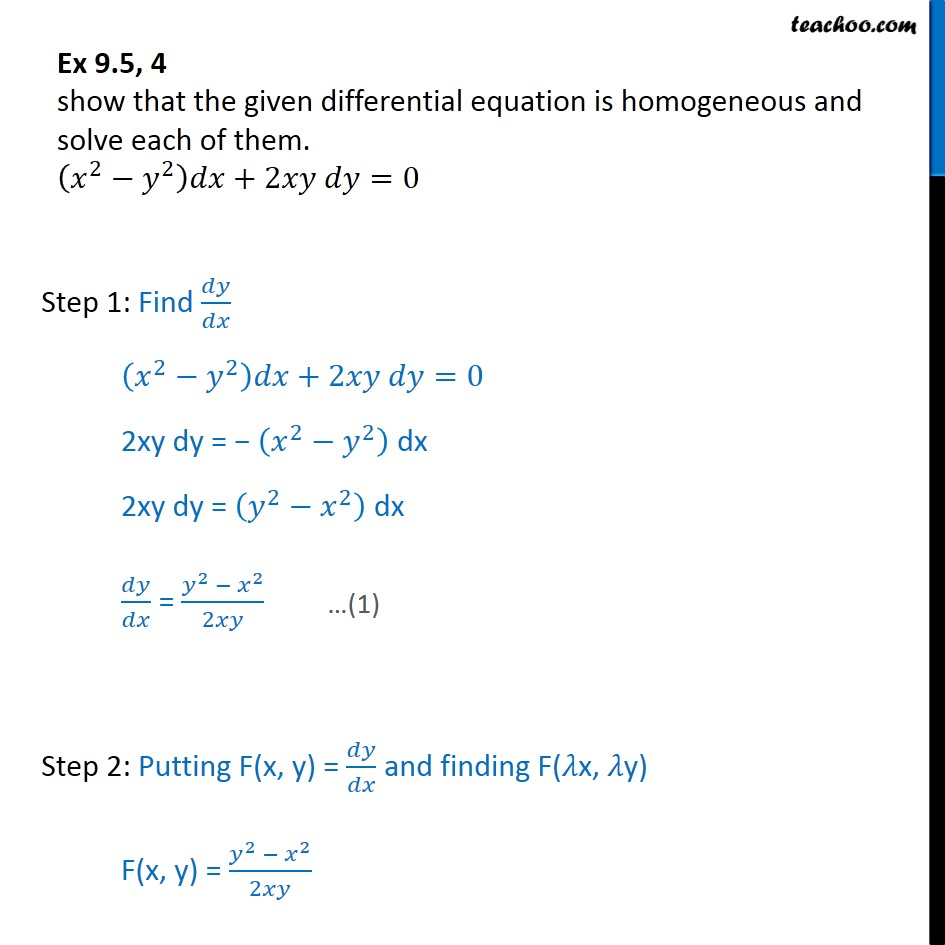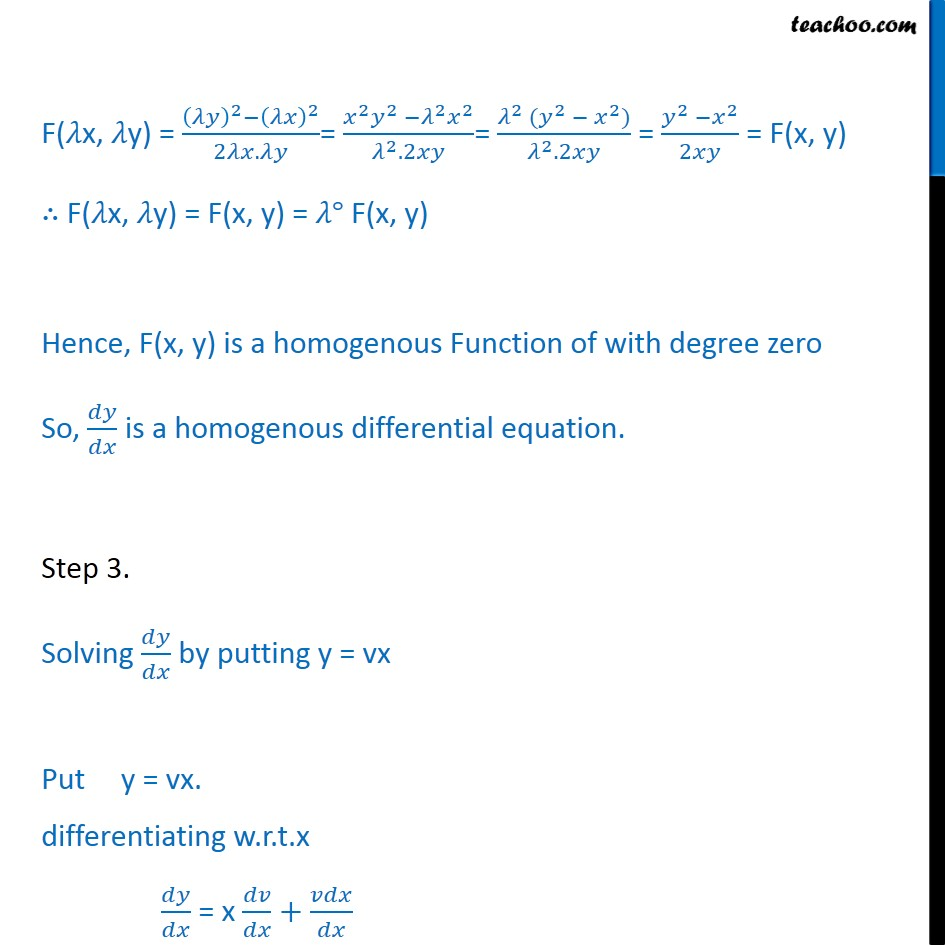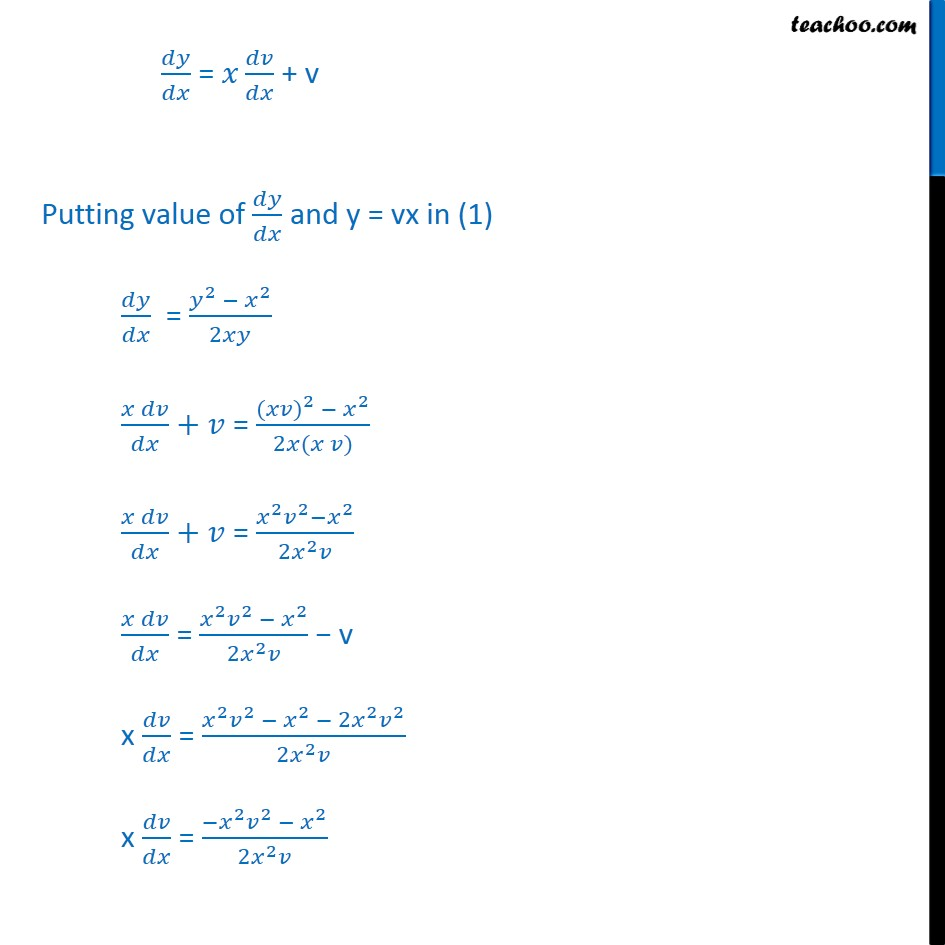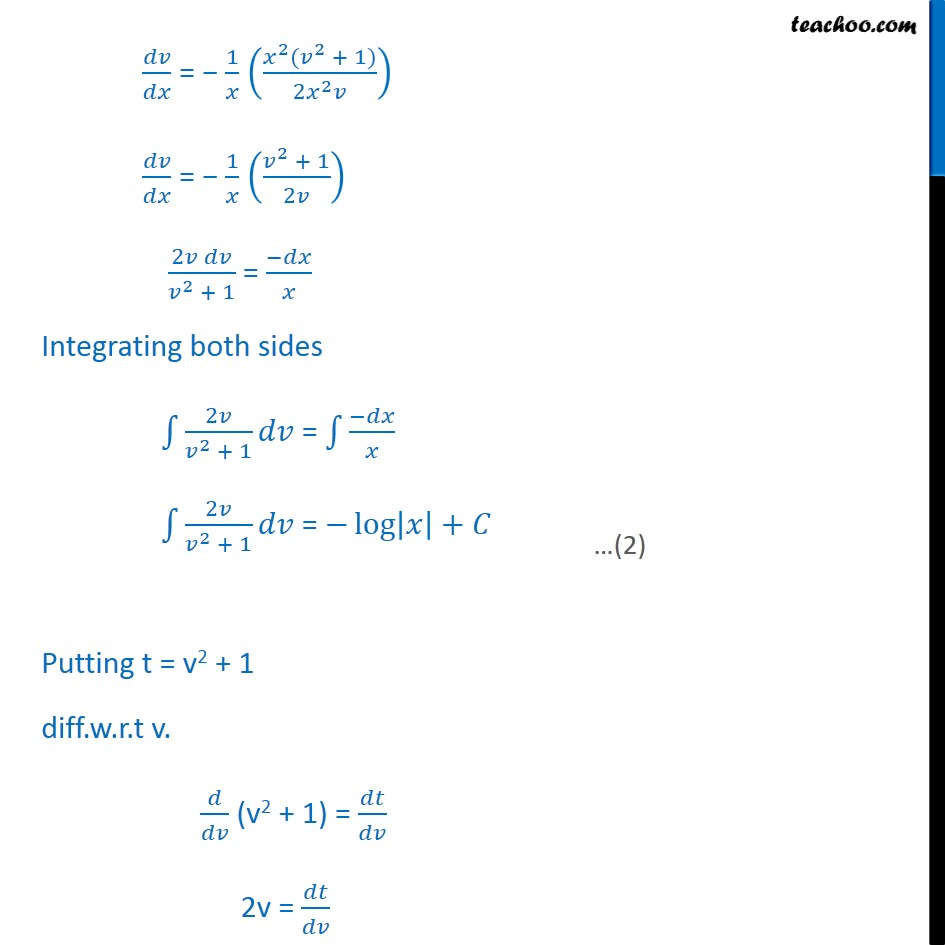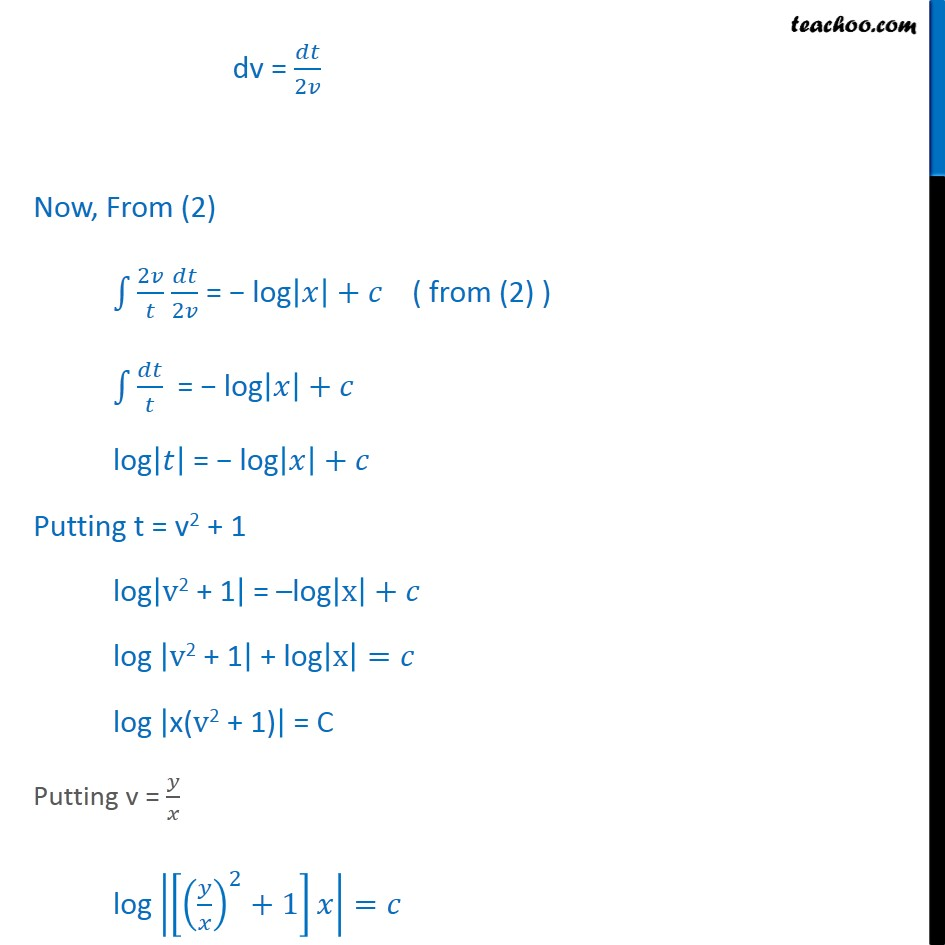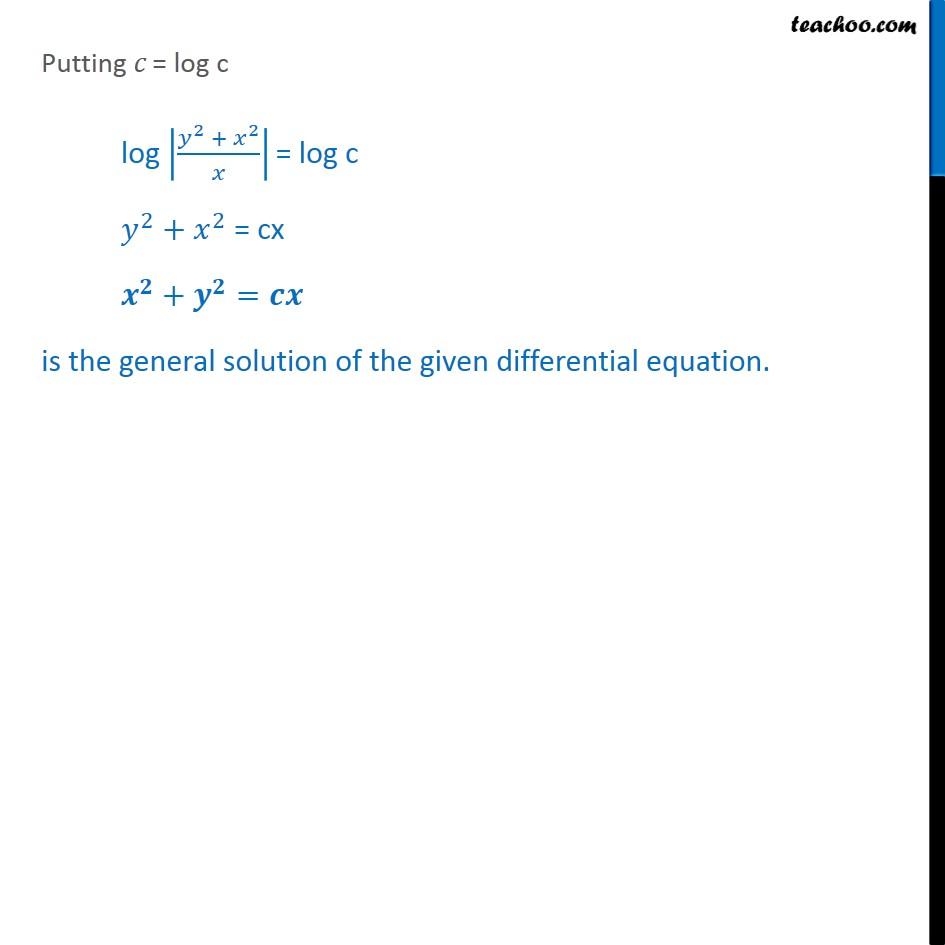Learn in your speed, with individual attention - Teachoo Maths 1-on-1 Class

### Transcript

Ex 9.4, 4 show that the given differential equation is homogeneous and solve each of them. ( ^2 ^2 ) +2 =0 Step 1: Find / ( ^2 ^2 ) +2 =0 2xy dy = ( ^2 ^2 ) dx 2xy dy = ( ^2 ^2 ) dx / = ( ^2 ^2)/2 Step 2: Putting F(x, y) = / and finding F( x, y) F(x, y) = ( ^2 ^2)/2 F( x, y) = (( )^2 ( )^2)/(2 . )= ( ^2 ^2 ^2 ^2)/( ^2.2 )= ( ^2 ( ^2 ^2))/( ^2.2 ) = ( ^2 ^2)/2 = F(x, y) F( x, y) = F(x, y) = F(x, y) Hence, F(x, y) is a homogenous Function of with degree zero So, / is a homogenous differential equation. Step 3. Solving / by putting y = vx Put y = vx. differentiating w.r.t.x / = x / + / / = / + v Putting value of / and y = vx in (1) / = ( ^2 ^2)/2 ( )/ + = ( ( ) ^2 ^2)/(2 ( )) ( )/ + = ( ^2 ^2 ^2)/(2 ^2 ) ( )/ = ( ^2 ^2 ^2)/(2 ^2 ) v x / = ( ^2 ^2 ^2 2 ^2 ^2)/(2 ^2 ) x / = ( ^2 ^2 ^2)/(2 ^2 ) / = 1/ (( ^2 ( ^2 + 1))/(2 ^2 )) / = 1/ (( ^2 + 1)/2 ) (2 )/( ^2 + 1) = ( )/ Integrating both sides 1 2 /( ^2 + 1) = 1 ( )/ 1 2 /( ^2 + 1) = log | |+ Putting t = v2 + 1 diff.w.r.t v. / (v2 + 1) = / 2v = / dv = /2 Now, From (2) 1 2 / /2 = log| |+ ( from (2) ) 1 / = log| |+ log| | = log| |+ Putting t = v2 + 1 log|"v2 + 1" | = log|"x" |+ log |"v2 + 1" | + log|"x" |= log |"x(v2 + 1)" | = C Putting v = / log |[( / )^2+1] |= log|( ^2 + ^2)/ ^2 |= Putting = log c log |( ^2 + ^2)/ | = log c ^2+ ^2 = cx ^ + ^ = is the general solution of the given differential equation.